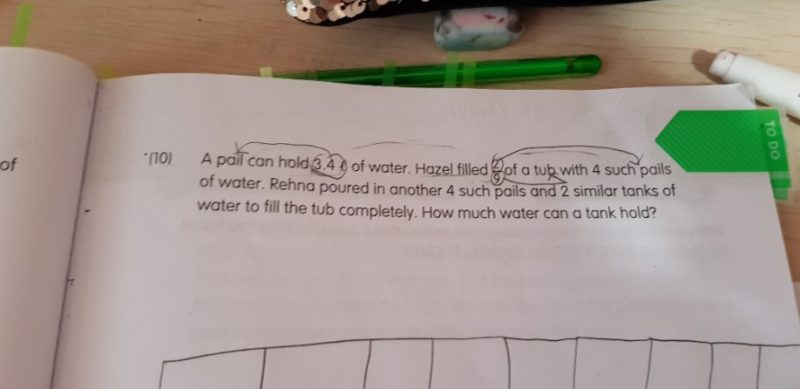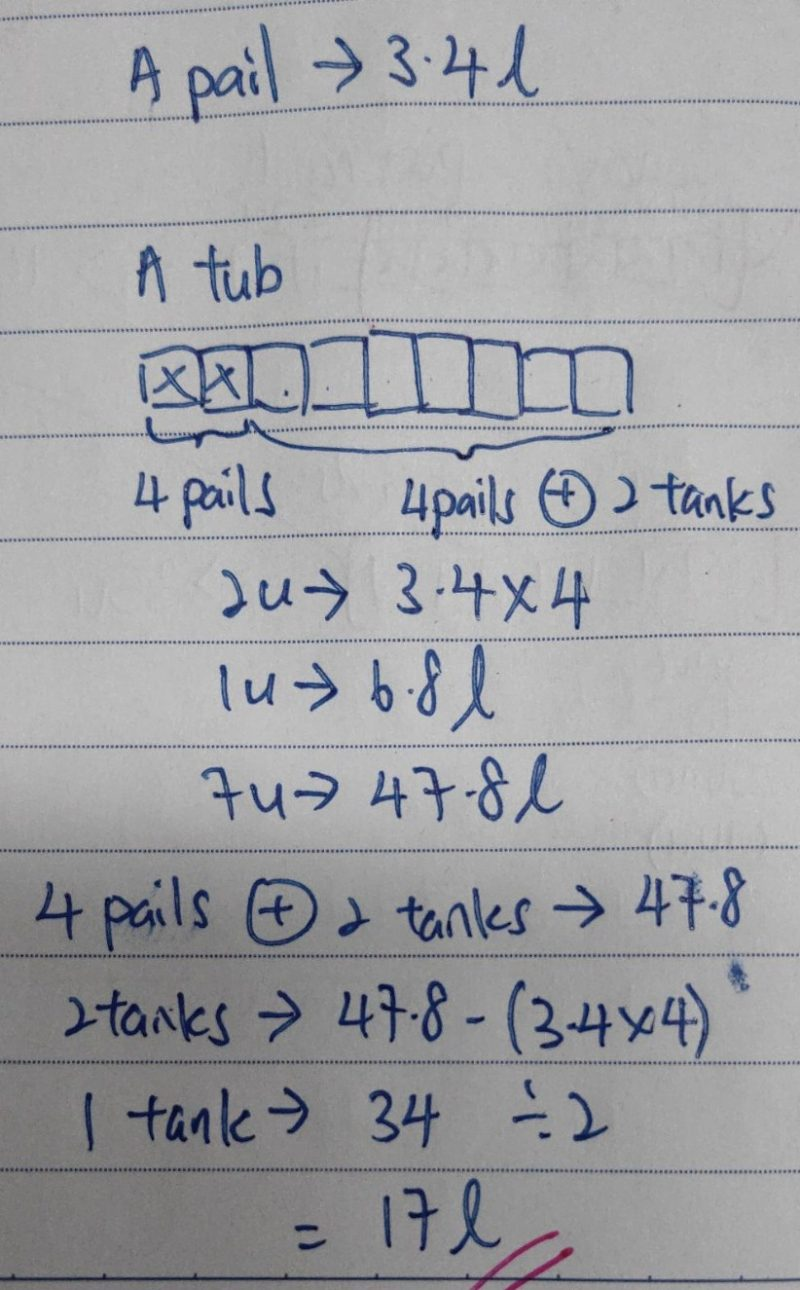QuestionP5. Fraction again. Thank you 😉1 Reply 0 Likes

Thank you. Its much more clearer with models. Have a nice day🤗

0 Replies 1 Like

volume of a pail = 3.4 lt

volume of 4 pails = 3.4 x 4 = 13.6 lt

2U= 13.6

9U = 9/2 x 13.6 = 4.5 x 13.6 = 61.2 lt

Volume of tub = 61.2 lt

Volume of tub = volume of 4 pails + volume of 4 pails + volume of 2 tanks

61.2 = 13.6 + 13.6   + volume of 2 tanks

volume of 2 tanks = 34 lt

volume of one tank = 17 litre.

Orckids Tuition, Ph: 8750 8449

0 Replies 0 Likes